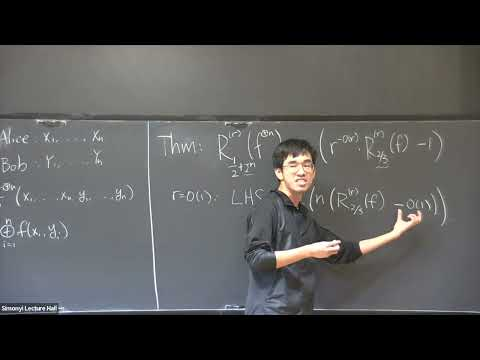### Videos

#### Strong XOR Lemma for Communication with Bounded Rounds

##### Presenter
November 21, 2022##### Abstract
In this talk, we show a strong XOR lemma for bounded-round two-player randomized communication.  For a function f:X×Y→{0,1}, the n-fold XOR function f^⊕n:X^n×Y^n→{0,1} maps n input pairs (x_1,...,x_n), (y_1,...,y_n) to the XOR of the n output bits f(x_1,y_1)⊕...⊕f(x_n, y_n).  We prove that if every r-round communication protocols that computes f with probability 2/3 uses at least C bits of communication, then any r-round protocol that computes f^⊕n with probability 1/2+exp(-O(n)) must use n(r^{-O (r)}C-1) bits.  When r is a constant and C is sufficiently large, this is Omega(nC) bits.  It matches the communication cost and the success probability of the trivial protocol that computes the n bits f(x_i,y_i) independently and outputs their XOR, up to a constant factor in n.   A similar XOR lemma has been proved for f whose communication lower bound can be obtained via bounding the discrepancy [Shaltiel03]. By the equivalence between the discrepancy and the correlation with 2-bit communication protocols, our new XOR lemma implies the previous result.